## Buildings.Fluid.Sensors

Package with sensor models

### Information

Package `Sensors` consists of idealized sensor components that provide variables of a medium as output signals. These signals can be, e.g., further processed with components of the Modelica.Blocks library.

Extends from Modelica.Icons.SensorsPackage (Icon for packages containing sensors).

### Package Content

NameDescriptionUsersGuide User's GuideConversions Package with conversions for sensor modelsDensity Ideal one port density sensorDensityTwoPort Ideal two port density sensorEnthalpyFlowRate Ideal enthalphy flow rate sensorSensibleEnthalpyFlowRate Ideal enthalphy flow rate sensor that outputs the sensible enthalpy flow rate onlyLatentEnthalpyFlowRate Ideal enthalphy flow rate sensor that outputs the latent enthalpy flow rate onlyMassFlowRate Ideal sensor for mass flow rateMassFraction Ideal one port mass fraction sensor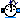MassFractionTwoPort Ideal two port mass fraction sensor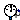Pressure Ideal pressure sensorRelativeHumidity Ideal one port relative humidity sensorRelativeHumidityTwoPort Ideal two port relative humidity sensorRelativePressure Ideal relative pressure sensorRelativeTemperature Ideal relative temperature sensorSpecificEnthalpy Ideal one port specific enthalpy sensorSpecificEnthalpyTwoPort Ideal two port sensor for the specific enthalpySpecificEntropy Ideal one port specific entropy sensorSpecificEntropyTwoPort Ideal two port sensor for the specific entropyTemperature Ideal one port temperature sensor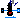TemperatureTwoPort Ideal two port temperature sensorTemperatureWetBulbTwoPort Ideal wet bulb temperature sensorTraceSubstances Ideal one port trace substances sensorTraceSubstancesTwoPort Ideal two port sensor for trace substanceVolumeFlowRate Ideal sensor for volume flow rate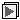Examples Collection of models that illustrate model use and test modelsBaseClasses Package with base classes for Buildings.Fluid.Sensors

##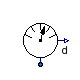Buildings.Fluid.Sensors.Density

Ideal one port density sensor### Information

This model outputs the density of the fluid connected to its port. The sensor is ideal, i.e. it does not influence the fluid.

Read the Buildings.Fluid.Sensors.UsersGuide prior to using this model with one fluid port.

Extends from Buildings.Fluid.Sensors.BaseClasses.PartialAbsoluteSensor (Partial component to model a sensor that measures a potential variable), Modelica.Icons.RotationalSensor (Icon representing a round measurement device).

### Parameters

TypeNameDefaultDescription
replaceable package MediumPartialMediumMedium in the sensor

### Connectors

TypeNameDescription
FluidPort_aport
output RealOutputdDensity in port medium [kg/m3]

### Modelica definition

```model Density "Ideal one port density sensor"
extends Buildings.Fluid.Sensors.BaseClasses.PartialAbsoluteSensor;
extends Modelica.Icons.RotationalSensor;
Modelica.Blocks.Interfaces.RealOutput d(final quantity="Density",
final unit="kg/m3",
min=0) "Density in port medium";

equation
d = Medium.density(
state=Medium.setState_phX(
p=port.p,
h=inStream(port.h_outflow),
X=inStream(port.Xi_outflow)));
end Density;
```

##Buildings.Fluid.Sensors.DensityTwoPort

Ideal two port density sensor### Information

This model outputs the density of the fluid flowing from `port_a` to `port_b`.

The sensor is ideal, i.e., it does not influence the fluid. If the parameter `tau` is non-zero, then its output is computed using a first order differential equation. Setting `tau=0` is not recommend. See Buildings.Fluid.Sensors.UsersGuide for an explanation.

Extends from Buildings.Fluid.Sensors.BaseClasses.PartialDynamicFlowSensor (Partial component to model sensors that measure flow properties using a dynamic model), Modelica.Icons.RotationalSensor (Icon representing a round measurement device).

### Parameters

TypeNameDefaultDescription
replaceable package MediumPartialMediumMedium in the component
Timetau1Time constant at nominal flow rate [s]
Nominal condition
MassFlowRatem_flow_nominal Nominal mass flow rate, used for regularization near zero flow [kg/s]
MassFlowRatem_flow_small1E-4*m_flow_nominalFor bi-directional flow, temperature is regularized in the region |m_flow| < m_flow_small (m_flow_small > 0 required) [kg/s]
Initialization
InitinitTypeModelica.Blocks.Types.Init.I...Type of initialization (InitialState and InitialOutput are identical)
Densityd_startMedium.density(state=Medium....Initial or guess value of output (=state) [kg/m3]
TemperatureT_startMedium.T_defaultTemperature used to compute d_start [K]
Pressurep_startMedium.p_defaultPressure used to compute d_start [Pa]
MassFractionX_start[Medium.nX]Medium.X_defaultMass fraction used to compute d_start 
Assumptions
BooleanallowFlowReversalsystem.allowFlowReversal= true to allow flow reversal, false restricts to design direction (port_a -> port_b)

### Connectors

TypeNameDescription
FluidPort_aport_aFluid connector a (positive design flow direction is from port_a to port_b)
FluidPort_bport_bFluid connector b (positive design flow direction is from port_a to port_b)
output RealOutputdDensity of the passing fluid [kg/m3]

### Modelica definition

```model DensityTwoPort "Ideal two port density sensor"
extends Buildings.Fluid.Sensors.BaseClasses.PartialDynamicFlowSensor;
extends Modelica.Icons.RotationalSensor;
Modelica.Blocks.Interfaces.RealOutput d(final quantity="Density",
final unit="kg/m3",
min=0) "Density of the passing fluid";
parameter Medium.Density d_start=
Medium.density(state=Medium.setState_pTX(
p=p_start, T=T_start, X=X_start))
"Initial or guess value of output (=state)";
parameter Modelica.SIunits.Temperature T_start=Medium.T_default
"Temperature used to compute d_start";
parameter Modelica.SIunits.Pressure p_start=Medium.p_default
"Pressure used to compute d_start";
parameter Modelica.SIunits.MassFraction X_start[Medium.nX]=Medium.X_default
"Mass fraction used to compute d_start";
protected
Medium.Density dMed(start=d_start)
"Medium density to which the sensor is exposed";

Medium.Density d_a_inflow "Density of inflowing fluid at port_a";
Medium.Density d_b_inflow
"Density of inflowing fluid at port_b, or rho_a_inflow if uni-directional flow";
initial equation
if dynamic then
der(d) = 0;
elseif initType == Modelica.Blocks.Types.Init.InitialState or
initType == Modelica.Blocks.Types.Init.InitialOutput then
d = d_start;
end if;
end if;
equation
if allowFlowReversal then
d_a_inflow = Medium.density(
state=Medium.setState_phX(p=port_b.p, h=port_b.h_outflow, X=port_b.Xi_outflow));
d_b_inflow = Medium.density(
state=Medium.setState_phX(p=port_a.p, h=port_a.h_outflow, X=port_a.Xi_outflow));
dMed = Modelica.Fluid.Utilities.regStep(
x=port_a.m_flow, y1=d_a_inflow, y2=d_b_inflow, x_small=m_flow_small);
else
dMed = Medium.density(
state=Medium.setState_phX(p=port_b.p, h=port_b.h_outflow, X=port_b.Xi_outflow));
d_a_inflow = dMed;
d_b_inflow = dMed;
end if;
// Output signal of sensor
if dynamic then
der(d) = (dMed-d)*k/tau;
else
d = dMed;
end if;
end DensityTwoPort;
```

##Buildings.Fluid.Sensors.EnthalpyFlowRate

Ideal enthalphy flow rate sensor### Information

This model outputs the enthalphy flow rate of the medium in the flow between fluid ports. The sensor is ideal, i.e., it does not influence the fluid.

If the parameter `tau` is non-zero, then the measured specific enthalpy hout that is used to compute the enthalpy flow rate Ḣ = ṁ hout is computed using a first order differential equation. See Buildings.Fluid.Sensors.UsersGuide for an explanation.

For a sensor that measures the latent enthalpy flow rate, use Buildings.Fluid.Sensors.LatentEnthalpyFlowRate.

Extends from Buildings.Fluid.Sensors.BaseClasses.PartialDynamicFlowSensor (Partial component to model sensors that measure flow properties using a dynamic model), Modelica.Icons.RotationalSensor (Icon representing a round measurement device).

### Parameters

TypeNameDefaultDescription
replaceable package MediumPartialMediumMedium in the component
Timetau1Time constant at nominal flow rate [s]
Nominal condition
MassFlowRatem_flow_nominal Nominal mass flow rate, used for regularization near zero flow [kg/s]
MassFlowRatem_flow_small1E-4*m_flow_nominalFor bi-directional flow, temperature is regularized in the region |m_flow| < m_flow_small (m_flow_small > 0 required) [kg/s]
Initialization
InitinitTypeModelica.Blocks.Types.Init.I...Type of initialization (InitialState and InitialOutput are identical)
SpecificEnthalpyh_out_startMedium.specificEnthalpy_pTX(...Initial or guess value of measured specific enthalpy [J/kg]
Assumptions
BooleanallowFlowReversalsystem.allowFlowReversal= true to allow flow reversal, false restricts to design direction (port_a -> port_b)

### Connectors

TypeNameDescription
FluidPort_aport_aFluid connector a (positive design flow direction is from port_a to port_b)
FluidPort_bport_bFluid connector b (positive design flow direction is from port_a to port_b)
output RealOutputH_flowEnthalpy flow rate, positive if from port_a to port_b [W]

### Modelica definition

```model EnthalpyFlowRate "Ideal enthalphy flow rate sensor"
extends Buildings.Fluid.Sensors.BaseClasses.PartialDynamicFlowSensor;
extends Modelica.Icons.RotationalSensor;
Modelica.Blocks.Interfaces.RealOutput H_flow(unit="W")
"Enthalpy flow rate, positive if from port_a to port_b";
parameter Modelica.SIunits.SpecificEnthalpy h_out_start=
Medium.specificEnthalpy_pTX(
p=Medium.p_default,
T=Medium.T_default,
X=Medium.X_default)
"Initial or guess value of measured specific enthalpy";
protected
Modelica.SIunits.SpecificEnthalpy hMed_out(start=h_out_start)
"Medium enthalpy to which the sensor is exposed";
Modelica.SIunits.SpecificEnthalpy h_out(start=h_out_start)
"Medium enthalpy that is used to compute the enthalpy flow rate";
initial equation
if dynamic then
der(h_out) = 0;
elseif initType == Modelica.Blocks.Types.Init.InitialState or
initType == Modelica.Blocks.Types.Init.InitialOutput then
h_out = h_out_start;
end if;
end if;
equation
if allowFlowReversal then
hMed_out = Modelica.Fluid.Utilities.regStep(
x=port_a.m_flow,
y1=port_b.h_outflow,
y2=port_a.h_outflow,
x_small=m_flow_small);
else
hMed_out = port_b.h_outflow;
end if;
// Specific enthalpy measured by sensor
if dynamic then
der(h_out) = (hMed_out-h_out)*k/tau;
else
h_out = hMed_out;
end if;
// Sensor output signal
H_flow = port_a.m_flow * h_out;
end EnthalpyFlowRate;
```

##Buildings.Fluid.Sensors.SensibleEnthalpyFlowRate

Ideal enthalphy flow rate sensor that outputs the sensible enthalpy flow rate only### Information

This model outputs the sensible enthalphy flow rate of the medium in the flow between its fluid ports. In particular, if the total enthalpy flow rate is

tot = Ḣsen + Ḣlat,

where sen = ṁ (1-Xw) cp,air, then this sensor outputs Ḣ = Ḣsen.

If the parameter `tau` is non-zero, then the measured specific sensible enthalpy hout that is used to compute the sensible enthalpy flow rate sen = ṁ hout is computed using a first order differential equation. See Buildings.Fluid.Sensors.UsersGuide for an explanation.

For a sensor that measures tot, use Buildings.Fluid.Sensors.EnthalpyFlowRate.
For a sensor that measures lat, use Buildings.Fluid.Sensors.LatentEnthalpyFlowRate.

The sensor is ideal, i.e., it does not influence the fluid. The sensor can only be used with medium models that implement the function `enthalpyOfNonCondensingGas(T)`.

Extends from Buildings.Fluid.Sensors.BaseClasses.PartialDynamicFlowSensor (Partial component to model sensors that measure flow properties using a dynamic model), Buildings.Fluid.BaseClasses.IndexMassFraction (Computes the index of a substance in the mass fraction vector Xi), Modelica.Icons.RotationalSensor (Icon representing a round measurement device).

### Parameters

TypeNameDefaultDescription
replaceable package MediumPartialMediumMedium in the component
Timetau1Time constant at nominal flow rate [s]
StringsubstanceName"water"Name of species substance
Nominal condition
MassFlowRatem_flow_nominal Nominal mass flow rate, used for regularization near zero flow [kg/s]
MassFlowRatem_flow_small1E-4*m_flow_nominalFor bi-directional flow, temperature is regularized in the region |m_flow| < m_flow_small (m_flow_small > 0 required) [kg/s]
Initialization
InitinitTypeModelica.Blocks.Types.Init.I...Type of initialization (InitialState and InitialOutput are identical)
SpecificEnthalpyh_out_startMedium.enthalpyOfNonCondensi...Initial or guess value of measured specific sensible enthalpy [J/kg]
Assumptions
BooleanallowFlowReversalsystem.allowFlowReversal= true to allow flow reversal, false restricts to design direction (port_a -> port_b)

### Connectors

TypeNameDescription
replaceable package MediumMedium in the component
FluidPort_aport_aFluid connector a (positive design flow direction is from port_a to port_b)
FluidPort_bport_bFluid connector b (positive design flow direction is from port_a to port_b)
output RealOutputH_flowSensible enthalpy flow rate, positive if from port_a to port_b [W]

### Modelica definition

```model SensibleEnthalpyFlowRate
"Ideal enthalphy flow rate sensor that outputs the sensible enthalpy flow rate only"
extends Buildings.Fluid.Sensors.BaseClasses.PartialDynamicFlowSensor(
redeclare replaceable package Medium =
Modelica.Media.Interfaces.PartialCondensingGases);
extends Buildings.Fluid.BaseClasses.IndexMassFraction(final substanceName="water");
extends Modelica.Icons.RotationalSensor;
Modelica.Blocks.Interfaces.RealOutput H_flow(final unit="W")
"Sensible enthalpy flow rate, positive if from port_a to port_b";
parameter Modelica.SIunits.SpecificEnthalpy h_out_start=
Medium.enthalpyOfNonCondensingGas(T=Medium.T_default)
"Initial or guess value of measured specific sensible enthalpy";
protected
Modelica.SIunits.SpecificEnthalpy hMed_out(start=h_out_start)
"Medium sensible enthalpy to which the sensor is exposed";
Modelica.SIunits.SpecificEnthalpy h_out(start=h_out_start)
"Medium sensible enthalpy that is used to compute the enthalpy flow rate";
Medium.MassFraction XiActual[Medium.nXi]
"Medium mass fraction to which sensor is exposed to";
Medium.SpecificEnthalpy hActual
"Medium enthalpy to which sensor is exposed to";
initial equation
// Compute initial state
if dynamic then
der(h_out) = 0;
elseif initType == Modelica.Blocks.Types.Init.InitialState or
initType == Modelica.Blocks.Types.Init.InitialOutput then
h_out = h_out_start;
end if;
end if;
equation
if allowFlowReversal then
XiActual = Modelica.Fluid.Utilities.regStep(
x=port_a.m_flow,
y1=port_b.Xi_outflow,
y2=port_a.Xi_outflow,
x_small=m_flow_small);
hActual = Modelica.Fluid.Utilities.regStep(
x=port_a.m_flow,
y1=port_b.h_outflow,
y2=port_a.h_outflow,
x_small=m_flow_small);
else
XiActual = port_b.Xi_outflow;
hActual = port_b.h_outflow;
end if;
// Specific enthalpy measured by sensor
hMed_out = (1-XiActual[i_x]) * Medium.enthalpyOfNonCondensingGas(
T=Medium.temperature(state=Medium.setState_phX(p=port_a.p, h=hActual, X=XiActual)));
if dynamic then
der(h_out) = (hMed_out-h_out)*k/tau;
else
h_out = hMed_out;
end if;
// Sensor output signal
H_flow = port_a.m_flow * h_out;
end SensibleEnthalpyFlowRate;
```

##Buildings.Fluid.Sensors.LatentEnthalpyFlowRate

Ideal enthalphy flow rate sensor that outputs the latent enthalpy flow rate only### Information

This model outputs the latent enthalphy flow rate of the medium in the flow between its fluid ports. In particular, if the total enthalpy flow rate is

tot = Ḣsen + Ḣlat,

where sen = ṁ (1-Xw) cp,air, then this sensor outputs Ḣ = Ḣlat.

If the parameter `tau` is non-zero, then the measured specific latent enthalpy hout that is used to compute the latent enthalpy flow rate lat = ṁ hout is computed using a first order differential equation. See Buildings.Fluid.Sensors.UsersGuide for an explanation.

For a sensor that measures tot, use Buildings.Fluid.Sensors.EnthalpyFlowRate.
For a sensor that measures sen, use Buildings.Fluid.Sensors.SensibleEnthalpyFlowRate.

The sensor is ideal, i.e., it does not influence the fluid. The sensor can only be used with medium models that implement the function `enthalpyOfNonCondensingGas(T)`.

Extends from Buildings.Fluid.Sensors.BaseClasses.PartialDynamicFlowSensor (Partial component to model sensors that measure flow properties using a dynamic model), Buildings.Fluid.BaseClasses.IndexMassFraction (Computes the index of a substance in the mass fraction vector Xi), Modelica.Icons.RotationalSensor (Icon representing a round measurement device).

### Parameters

TypeNameDefaultDescription
replaceable package MediumPartialMediumMedium in the component
Timetau1Time constant at nominal flow rate [s]
StringsubstanceName"water"Name of species substance
Nominal condition
MassFlowRatem_flow_nominal Nominal mass flow rate, used for regularization near zero flow [kg/s]
MassFlowRatem_flow_small1E-4*m_flow_nominalFor bi-directional flow, temperature is regularized in the region |m_flow| < m_flow_small (m_flow_small > 0 required) [kg/s]
Initialization
InitinitTypeModelica.Blocks.Types.Init.I...Type of initialization (InitialState and InitialOutput are identical)
SpecificEnthalpyh_out_startMedium.specificEnthalpy_pTX(...Initial or guess value of measured specific latent enthalpy [J/kg]
Assumptions
BooleanallowFlowReversalsystem.allowFlowReversal= true to allow flow reversal, false restricts to design direction (port_a -> port_b)

### Connectors

TypeNameDescription
replaceable package MediumMedium in the component
FluidPort_aport_aFluid connector a (positive design flow direction is from port_a to port_b)
FluidPort_bport_bFluid connector b (positive design flow direction is from port_a to port_b)
output RealOutputH_flowLatent enthalpy flow rate, positive if from port_a to port_b [W]

### Modelica definition

```model LatentEnthalpyFlowRate
"Ideal enthalphy flow rate sensor that outputs the latent enthalpy flow rate only"
extends Buildings.Fluid.Sensors.BaseClasses.PartialDynamicFlowSensor(
redeclare replaceable package Medium =
Modelica.Media.Interfaces.PartialCondensingGases);
extends Buildings.Fluid.BaseClasses.IndexMassFraction(final substanceName="water");
extends Modelica.Icons.RotationalSensor;
Modelica.Blocks.Interfaces.RealOutput H_flow(final unit="W")
"Latent enthalpy flow rate, positive if from port_a to port_b";
parameter Modelica.SIunits.SpecificEnthalpy h_out_start=
Medium.specificEnthalpy_pTX(
p=Medium.p_default, T=Medium.T_default, X=Medium.X_default)
-Medium.enthalpyOfNonCondensingGas(T=Medium.T_default)
"Initial or guess value of measured specific latent enthalpy";

protected
Modelica.SIunits.SpecificEnthalpy hMed_out(start=h_out_start)
"Medium latent enthalpy to which the sensor is exposed";
Modelica.SIunits.SpecificEnthalpy h_out(start=h_out_start)
"Medium latent enthalpy that is used to compute the enthalpy flow rate";

Medium.MassFraction XiActual[Medium.nXi]
"Medium mass fraction to which sensor is exposed to";
Medium.SpecificEnthalpy hActual
"Medium enthalpy to which sensor is exposed to";
initial equation
// Compute initial state
if dynamic then
der(h_out) = 0;
elseif initType == Modelica.Blocks.Types.Init.InitialState or
initType == Modelica.Blocks.Types.Init.InitialOutput then
h_out = h_out_start;
end if;
end if;
equation
if allowFlowReversal then
XiActual = Modelica.Fluid.Utilities.regStep(
x=port_a.m_flow,
y1=port_b.Xi_outflow,
y2=port_a.Xi_outflow,
x_small=m_flow_small);
hActual = Modelica.Fluid.Utilities.regStep(
x=port_a.m_flow,
y1=port_b.h_outflow,
y2=port_a.h_outflow,
x_small=m_flow_small);
else
XiActual = port_b.Xi_outflow;
hActual = port_b.h_outflow;
end if;
// Specific enthalpy measured by sensor.
// Compute H_flow as difference between total enthalpy and enthalpy on non-condensing gas.
// This is needed to compute the liquid vs. gas fraction of water, using the equations
// provided by the medium model
hMed_out = (hActual -
(1-XiActual[i_x]) * Medium.enthalpyOfNonCondensingGas(
T=Medium.temperature(Medium.setState_phX(p=port_a.p, h=hActual, X=XiActual))));
if dynamic then
der(h_out) = (hMed_out-h_out)*k/tau;
else
h_out = hMed_out;
end if;
// Sensor output signal
H_flow = port_a.m_flow * h_out;
end LatentEnthalpyFlowRate;
```

##Buildings.Fluid.Sensors.MassFlowRate

Ideal sensor for mass flow rate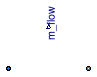### Information

This model outputs the mass flow rate flowing from `port_a` to `port_b`. The sensor is ideal, i.e., it does not influence the fluid.

Extends from Buildings.Fluid.Sensors.BaseClasses.PartialFlowSensor (Partial component to model sensors that measure flow properties), Modelica.Icons.RotationalSensor (Icon representing a round measurement device).

### Parameters

TypeNameDefaultDescription
replaceable package MediumPartialMediumMedium in the component
Nominal condition
MassFlowRatem_flow_nominal0Nominal mass flow rate, used for regularization near zero flow [kg/s]
MassFlowRatem_flow_small0For bi-directional flow, temperature is regularized in the region |m_flow| < m_flow_small (m_flow_small > 0 required) [kg/s]
Assumptions
BooleanallowFlowReversalsystem.allowFlowReversal= true to allow flow reversal, false restricts to design direction (port_a -> port_b)

### Connectors

TypeNameDescription
FluidPort_aport_aFluid connector a (positive design flow direction is from port_a to port_b)
FluidPort_bport_bFluid connector b (positive design flow direction is from port_a to port_b)
output RealOutputm_flowMass flow rate from port_a to port_b [kg/s]

### Modelica definition

```model MassFlowRate "Ideal sensor for mass flow rate"
extends Buildings.Fluid.Sensors.BaseClasses.PartialFlowSensor(
final m_flow_nominal = 0,
final m_flow_small = 0);
extends Modelica.Icons.RotationalSensor;
Modelica.Blocks.Interfaces.RealOutput m_flow(quantity="MassFlowRate",
final unit="kg/s")
"Mass flow rate from port_a to port_b";

equation
m_flow = port_a.m_flow;
end MassFlowRate;
```

##Buildings.Fluid.Sensors.MassFraction

Ideal one port mass fraction sensor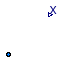### Information

This model outputs the mass fraction of the fluid connected to its port. The sensor is ideal, i.e., it does not influence the fluid.

Read the Buildings.Fluid.Sensors.UsersGuide prior to using this model with one fluid port.

Extends from Buildings.Fluid.Sensors.BaseClasses.PartialAbsoluteSensor (Partial component to model a sensor that measures a potential variable), Buildings.Fluid.BaseClasses.IndexMassFraction (Computes the index of a substance in the mass fraction vector Xi), Modelica.Icons.RotationalSensor (Icon representing a round measurement device).

### Parameters

TypeNameDefaultDescription
replaceable package MediumPartialMediumMedium in the sensor
StringsubstanceName"water"Name of species substance

### Connectors

TypeNameDescription
replaceable package MediumMedium in the sensor
FluidPort_aport
output RealOutputXMass fraction in port 

### Modelica definition

```model MassFraction "Ideal one port mass fraction sensor"
extends Buildings.Fluid.Sensors.BaseClasses.PartialAbsoluteSensor(
redeclare replaceable package Medium =
Modelica.Media.Interfaces.PartialCondensingGases);
extends Buildings.Fluid.BaseClasses.IndexMassFraction(substanceName = "water");
extends Modelica.Icons.RotationalSensor;

Modelica.Blocks.Interfaces.RealOutput X(min=-1e-3,
max=1.001,
unit="1") "Mass fraction in port";
protected
Medium.MassFraction XiVec[Medium.nXi](
quantity=Medium.extraPropertiesNames)
"Mass fraction vector, needed because indexed argument for the operator inStream is not supported";

equation
XiVec = inStream(port.Xi_outflow);
X = if i_x > Medium.nXi then (1-sum(XiVec)) else XiVec[i_x];
end MassFraction;
```

##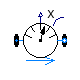Buildings.Fluid.Sensors.MassFractionTwoPort

Ideal two port mass fraction sensor### Information

This model outputs the mass fraction of the passing fluid. The sensor is ideal, i.e. it does not influence the fluid. If the parameter `tau` is non-zero, then its output is computed using a first order differential equation. Setting `tau=0` is not recommend. See Buildings.Fluid.Sensors.UsersGuide for an explanation.

Extends from Buildings.Fluid.Sensors.BaseClasses.PartialDynamicFlowSensor (Partial component to model sensors that measure flow properties using a dynamic model), Buildings.Fluid.BaseClasses.IndexMassFraction (Computes the index of a substance in the mass fraction vector Xi), Modelica.Icons.RotationalSensor (Icon representing a round measurement device).

### Parameters

TypeNameDefaultDescription
replaceable package MediumPartialMediumMedium in the component
Timetau1Time constant at nominal flow rate [s]
StringsubstanceName"water"Name of species substance
Nominal condition
MassFlowRatem_flow_nominal Nominal mass flow rate, used for regularization near zero flow [kg/s]
MassFlowRatem_flow_small1E-4*m_flow_nominalFor bi-directional flow, temperature is regularized in the region |m_flow| < m_flow_small (m_flow_small > 0 required) [kg/s]
Initialization
InitinitTypeModelica.Blocks.Types.Init.I...Type of initialization (InitialState and InitialOutput are identical)
MassFractionX_startMedium.X_default[i_x]Initial or guess value of output (= state) [kg/kg]
Assumptions
BooleanallowFlowReversalsystem.allowFlowReversal= true to allow flow reversal, false restricts to design direction (port_a -> port_b)

### Connectors

TypeNameDescription
replaceable package MediumMedium in the component
FluidPort_aport_aFluid connector a (positive design flow direction is from port_a to port_b)
FluidPort_bport_bFluid connector b (positive design flow direction is from port_a to port_b)
output RealOutputXMass fraction of the passing fluid 

### Modelica definition

```model MassFractionTwoPort "Ideal two port mass fraction sensor"
extends Buildings.Fluid.Sensors.BaseClasses.PartialDynamicFlowSensor(
redeclare replaceable package Medium =
Modelica.Media.Interfaces.PartialCondensingGases);
extends Buildings.Fluid.BaseClasses.IndexMassFraction(substanceName = "water");
extends Modelica.Icons.RotationalSensor;

parameter Medium.MassFraction X_start=Medium.X_default[i_x]
"Initial or guess value of output (= state)";

Modelica.Blocks.Interfaces.RealOutput X(min=-1e-3,
max=1.001,
start=X_start,
unit="1")
"Mass fraction of the passing fluid";

protected
Medium.MassFraction XMed(start=X_start)
"Mass fraction to which the sensor is exposed";
Medium.MassFraction XiVec[Medium.nXi](
quantity=Medium.extraPropertiesNames)
"Trace substances vector, needed because indexed argument for the operator inStream is not supported";
initial equation
if dynamic then
der(X) = 0;
elseif initType == Modelica.Blocks.Types.Init.InitialState or
initType == Modelica.Blocks.Types.Init.InitialOutput then
X = X_start;
end if;
end if;
equation
if allowFlowReversal then
XiVec = Modelica.Fluid.Utilities.regStep(
x=port_a.m_flow,
y1=port_b.Xi_outflow,
y2=port_a.Xi_outflow,
x_small=m_flow_small);
else
XiVec = port_b.Xi_outflow;
end if;
XMed = if i_x > Medium.nXi then (1-sum(XiVec)) else XiVec[i_x];
// Output signal of sensor
if dynamic then
der(X)  = (XMed-X)*k/tau;
else
X = XMed;
end if;
end MassFractionTwoPort;
```

##Buildings.Fluid.Sensors.Pressure

Ideal pressure sensor### Information

This model outputs the absolute pressure of the fluid connected to its port. The sensor is ideal, i.e., it does not influence the fluid.

Extends from Buildings.Fluid.Sensors.BaseClasses.PartialAbsoluteSensor (Partial component to model a sensor that measures a potential variable), Modelica.Icons.RotationalSensor (Icon representing a round measurement device).

### Parameters

TypeNameDefaultDescription
replaceable package MediumPartialMediumMedium in the sensor

### Connectors

TypeNameDescription
FluidPort_aport
output RealOutputpPressure at port [Pa]

### Modelica definition

```model Pressure "Ideal pressure sensor"
extends Buildings.Fluid.Sensors.BaseClasses.PartialAbsoluteSensor;
extends Modelica.Icons.RotationalSensor;
Modelica.Blocks.Interfaces.RealOutput p(final quantity="Pressure",
final unit="Pa",
min=0) "Pressure at port";
equation
p = port.p;
end Pressure;
```

##Buildings.Fluid.Sensors.RelativeHumidity

Ideal one port relative humidity sensor### Information

This model outputs the relative humidity of the fluid connected to its port. The sensor is ideal, i.e. it does not influence the fluid.

Note that this sensor can only be used with media that contain the variable `phi`, which is typically the case for moist air models.

Read the Buildings.Fluid.Sensors.UsersGuide prior to using this model with one fluid port.

Extends from Buildings.Fluid.Sensors.BaseClasses.PartialAbsoluteSensor (Partial component to model a sensor that measures a potential variable), Modelica.Icons.RotationalSensor (Icon representing a round measurement device).

### Parameters

TypeNameDefaultDescription
replaceable package MediumPartialMediumMedium in the sensor

### Connectors

TypeNameDescription
FluidPort_aport
output RealOutputphiRelative humidity in port medium 

### Modelica definition

```model RelativeHumidity "Ideal one port relative humidity sensor"
extends Buildings.Fluid.Sensors.BaseClasses.PartialAbsoluteSensor;
extends Modelica.Icons.RotationalSensor;

Modelica.Blocks.Interfaces.RealOutput phi(unit="1", min=0)
"Relative humidity in port medium";

protected
Medium.BaseProperties med "Medium state at dry bulb temperature";

equation
med.p = port.p;
med.h = inStream(port.h_outflow);
med.Xi = inStream(port.Xi_outflow);
phi = med.phi;

end RelativeHumidity;
```

##Buildings.Fluid.Sensors.RelativeHumidityTwoPort

Ideal two port relative humidity sensor### Information

This model outputs the relative humidity of the fluid flowing from `port_a` to `port_b`. The sensor is ideal, i.e., it does not influence the fluid.

Note that this sensor can only be used with media that contain the variable `phi`, which is typically the case for moist air models.

If the parameter `tau` is non-zero, then its output is computed using a first order differential equation. Setting `tau=0` is not recommend. See Buildings.Fluid.Sensors.UsersGuide for an explanation.

Extends from Buildings.Fluid.Sensors.BaseClasses.PartialDynamicFlowSensor (Partial component to model sensors that measure flow properties using a dynamic model), Modelica.Icons.RotationalSensor (Icon representing a round measurement device).

### Parameters

TypeNameDefaultDescription
replaceable package MediumPartialMediumMedium in the component
Timetau1Time constant at nominal flow rate [s]
Nominal condition
MassFlowRatem_flow_nominal Nominal mass flow rate, used for regularization near zero flow [kg/s]
MassFlowRatem_flow_small1E-4*m_flow_nominalFor bi-directional flow, temperature is regularized in the region |m_flow| < m_flow_small (m_flow_small > 0 required) [kg/s]
Initialization
InitinitTypeModelica.Blocks.Types.Init.I...Type of initialization (InitialState and InitialOutput are identical)
Realphi_start0.5Initial or guess value of output (= state) 
Assumptions
BooleanallowFlowReversalsystem.allowFlowReversal= true to allow flow reversal, false restricts to design direction (port_a -> port_b)

### Connectors

TypeNameDescription
FluidPort_aport_aFluid connector a (positive design flow direction is from port_a to port_b)
FluidPort_bport_bFluid connector b (positive design flow direction is from port_a to port_b)
output RealOutputphiRelative humidity of the passing fluid 

### Modelica definition

```model RelativeHumidityTwoPort
"Ideal two port relative humidity sensor"
extends Buildings.Fluid.Sensors.BaseClasses.PartialDynamicFlowSensor;
extends Modelica.Icons.RotationalSensor;
Modelica.Blocks.Interfaces.RealOutput phi(unit="1",
min=0,
start=phi_start)
"Relative humidity of the passing fluid";
parameter Real phi_start(unit="1", min=0, max=1)=0.5
"Initial or guess value of output (= state)";

protected
Real phiMed(unit="1", min=0, start=phi_start)
"Relative humidity to which the sensor is exposed to";
Medium.BaseProperties med_a_inflow
"Medium state of inflowing fluid at port a";
Medium.BaseProperties med_b_inflow
"Medium state of inflowing fluid at port b";
initial equation
if dynamic then
der(phi) = 0;
elseif initType == Modelica.Blocks.Types.Init.InitialState or
initType == Modelica.Blocks.Types.Init.InitialOutput then
phi = phi_start;
end if;
end if;
equation
med_a_inflow.p  = port_a.p;
med_a_inflow.h  = port_b.h_outflow;
med_a_inflow.Xi = port_b.Xi_outflow;
med_b_inflow.p  = port_b.p;
med_b_inflow.h  = port_a.h_outflow;
med_b_inflow.Xi = port_a.Xi_outflow;
if allowFlowReversal then
phiMed = Modelica.Fluid.Utilities.regStep(
x=port_a.m_flow,
y1=med_a_inflow.phi,
y2=med_b_inflow.phi,
x_small=m_flow_small);
else
phiMed = med_a_inflow.phi;
end if;
// Output signal of sensor
if dynamic then
der(phi) = (phiMed-phi)*k/tau;
else
phi = phiMed;
end if;
end RelativeHumidityTwoPort;
```

##Buildings.Fluid.Sensors.RelativePressure

Ideal relative pressure sensor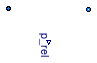### Information

The relative pressure `port_a.p - port_b.p` is determined between the two ports of this component and is provided as output signal. The sensor should be connected in parallel with other equipment, no flow through the sensor is allowed.

Extends from Modelica.Icons.TranslationalSensor (Icon representing a linear measurement device).

### Parameters

TypeNameDefaultDescription
replaceable package MediumModelica.Media.Interfaces.Pa...Medium in the sensor

### Connectors

TypeNameDescription
replaceable package MediumMedium in the sensor
FluidPort_aport_aFluid connector of stream a
FluidPort_bport_bFluid connector of stream b
output RealOutputp_relRelative pressure of port_a minus port_b [Pa]

### Modelica definition

```model RelativePressure "Ideal relative pressure sensor"
extends Modelica.Icons.TranslationalSensor;
replaceable package Medium =
Modelica.Media.Interfaces.PartialMedium "Medium in the sensor";

Modelica.Fluid.Interfaces.FluidPort_a port_a(m_flow(min=0),
p(start=Medium.p_default),
redeclare package Medium = Medium)
"Fluid connector of stream a";
Modelica.Fluid.Interfaces.FluidPort_b port_b(m_flow(min=0),
p(start=Medium.p_default),
redeclare package Medium = Medium)
"Fluid connector of stream b";

Modelica.Blocks.Interfaces.RealOutput p_rel(final quantity="Pressure",
final unit="Pa",
displayUnit="Pa")
"Relative pressure of port_a minus port_b";
equation
// Zero flow equations for connectors
port_a.m_flow = 0;
port_b.m_flow = 0;

// No contribution of specific quantities
port_a.h_outflow = 0;
port_b.h_outflow = 0;
port_a.Xi_outflow = zeros(Medium.nXi);
port_b.Xi_outflow = zeros(Medium.nXi);
port_a.C_outflow  = zeros(Medium.nC);
port_b.C_outflow  = zeros(Medium.nC);

// Relative pressure
p_rel = port_a.p - port_b.p;
end RelativePressure;
```

##Buildings.Fluid.Sensors.RelativeTemperature

Ideal relative temperature sensor### Information

The relative temperature `T(port_a) - T(port_b)` is determined between the two ports of this component and is provided as output signal. The sensor should be connected in parallel with other equipment. There is no flow through the sensor.

Note that this sensor should only be connected to fluid volumes, such as Buildings.Fluid.MixingVolumes.MixingVolume. Otherwise, numerical problems may occur if one of the mass flow rates are close to zero. See Buildings.Fluid.Sensors.UsersGuide for an explanation.

Extends from Modelica.Icons.TranslationalSensor (Icon representing a linear measurement device).

### Parameters

TypeNameDefaultDescription
replaceable package MediumModelica.Media.Interfaces.Pa...Medium in the sensor

### Connectors

TypeNameDescription
replaceable package MediumMedium in the sensor
FluidPort_aport_aFluid connector of stream a
FluidPort_bport_bFluid connector of stream b
output RealOutputT_relTemperature difference of port_a minus port_b [K]

### Modelica definition

```model RelativeTemperature "Ideal relative temperature sensor"
extends Modelica.Icons.TranslationalSensor;
replaceable package Medium =
Modelica.Media.Interfaces.PartialMedium "Medium in the sensor";
Modelica.Fluid.Interfaces.FluidPort_a port_a(m_flow(min=0),
p(start=Medium.p_default),
redeclare package Medium = Medium)
"Fluid connector of stream a";
Modelica.Fluid.Interfaces.FluidPort_b port_b(m_flow(min=0),
p(start=Medium.p_default),
redeclare package Medium = Medium)
"Fluid connector of stream b";
Modelica.Blocks.Interfaces.RealOutput T_rel(final unit = "K",
displayUnit = "K")
"Temperature difference of port_a minus port_b";
equation
// Zero flow equations for connectors
port_a.m_flow = 0;
port_b.m_flow = 0;
// No contribution of specific quantities
port_a.h_outflow = 0;
port_b.h_outflow = 0;
port_a.Xi_outflow = zeros(Medium.nXi);
port_b.Xi_outflow = zeros(Medium.nXi);
port_a.C_outflow  = zeros(Medium.nC);
port_b.C_outflow  = zeros(Medium.nC);
// Relative temperature
T_rel = Medium.temperature(state=Medium.setState_phX(
p=port_a.p, h=inStream(port_a.h_outflow), X=inStream(port_a.Xi_outflow))) -
Medium.temperature(state=Medium.setState_phX(
p=port_b.p, h=inStream(port_b.h_outflow), X=inStream(port_b.Xi_outflow)));
end RelativeTemperature;
```

##Buildings.Fluid.Sensors.SpecificEnthalpy

Ideal one port specific enthalpy sensor### Information

This model outputs the specific enthalpy of the fluid connected to its port. The sensor is ideal, i.e. it does not influence the fluid.

Read the Buildings.Fluid.Sensors.UsersGuide prior to using this model with one fluid port.

Extends from Buildings.Fluid.Sensors.BaseClasses.PartialAbsoluteSensor (Partial component to model a sensor that measures a potential variable), Modelica.Icons.RotationalSensor (Icon representing a round measurement device).

### Parameters

TypeNameDefaultDescription
replaceable package MediumPartialMediumMedium in the sensor

### Connectors

TypeNameDescription
FluidPort_aport
output RealOutputh_outSpecific enthalpy in port medium [J/kg]

### Modelica definition

```model SpecificEnthalpy "Ideal one port specific enthalpy sensor"
extends Buildings.Fluid.Sensors.BaseClasses.PartialAbsoluteSensor;
extends Modelica.Icons.RotationalSensor;
Modelica.Blocks.Interfaces.RealOutput h_out(final quantity="SpecificEnergy",
final unit="J/kg")
"Specific enthalpy in port medium";
equation
h_out = inStream(port.h_outflow);
end SpecificEnthalpy;
```

##Buildings.Fluid.Sensors.SpecificEnthalpyTwoPort

Ideal two port sensor for the specific enthalpy### Information

This model outputs the specific enthalpy of a passing fluid. The sensor is ideal, i.e. it does not influence the fluid. If the parameter `tau` is non-zero, then its output is computed using a first order differential equation. Setting `tau=0` is not recommend. See Buildings.Fluid.Sensors.UsersGuide for an explanation.

Extends from Buildings.Fluid.Sensors.BaseClasses.PartialDynamicFlowSensor (Partial component to model sensors that measure flow properties using a dynamic model), Modelica.Icons.RotationalSensor (Icon representing a round measurement device).

### Parameters

TypeNameDefaultDescription
replaceable package MediumPartialMediumMedium in the component
Timetau1Time constant at nominal flow rate [s]
Nominal condition
MassFlowRatem_flow_nominal Nominal mass flow rate, used for regularization near zero flow [kg/s]
MassFlowRatem_flow_small1E-4*m_flow_nominalFor bi-directional flow, temperature is regularized in the region |m_flow| < m_flow_small (m_flow_small > 0 required) [kg/s]
Initialization
InitinitTypeModelica.Blocks.Types.Init.I...Type of initialization (InitialState and InitialOutput are identical)
SpecificEnthalpyh_out_startMedium.specificEnthalpy_pTX(...Initial or guess value of output (= state) [J/kg]
Assumptions
BooleanallowFlowReversalsystem.allowFlowReversal= true to allow flow reversal, false restricts to design direction (port_a -> port_b)

### Connectors

TypeNameDescription
FluidPort_aport_aFluid connector a (positive design flow direction is from port_a to port_b)
FluidPort_bport_bFluid connector b (positive design flow direction is from port_a to port_b)
output RealOutputh_outSpecific enthalpy of the passing fluid [J/kg]

### Modelica definition

```model SpecificEnthalpyTwoPort
"Ideal two port sensor for the specific enthalpy"
extends Buildings.Fluid.Sensors.BaseClasses.PartialDynamicFlowSensor;
extends Modelica.Icons.RotationalSensor;
parameter Modelica.SIunits.SpecificEnthalpy h_out_start=
Medium.specificEnthalpy_pTX(p=Medium.p_default, T=Medium.T_default, X=Medium.X_default)
"Initial or guess value of output (= state)";
Modelica.Blocks.Interfaces.RealOutput h_out(final quantity="SpecificEnergy",
final unit="J/kg",
start=h_out_start)
"Specific enthalpy of the passing fluid";
protected
Modelica.SIunits.SpecificEnthalpy hMed_out(start=h_out_start)
"Medium enthalpy to which the sensor is exposed";
initial equation
if dynamic then
der(h_out) = 0;
elseif initType == Modelica.Blocks.Types.Init.InitialState or
initType == Modelica.Blocks.Types.Init.InitialOutput then
h_out = h_out_start;
end if;
end if;
equation
if allowFlowReversal then
hMed_out = Modelica.Fluid.Utilities.regStep(
x=port_a.m_flow,
y1=port_b.h_outflow,
y2=port_a.h_outflow,
x_small=m_flow_small);
else
hMed_out = port_b.h_outflow;
end if;
// Output signal of sensor
if dynamic then
der(h_out) = (hMed_out-h_out)*k/tau;
else
h_out = hMed_out;
end if;
end SpecificEnthalpyTwoPort;
```

##Buildings.Fluid.Sensors.SpecificEntropy

Ideal one port specific entropy sensor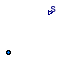### Information

This model outputs the specific entropy of the fluid connected to its port. The sensor is ideal, i.e., it does not influence the fluid.

Read the Buildings.Fluid.Sensors.UsersGuide prior to using this model with one fluid port.

Extends from Buildings.Fluid.Sensors.BaseClasses.PartialAbsoluteSensor (Partial component to model a sensor that measures a potential variable), Modelica.Icons.RotationalSensor (Icon representing a round measurement device).

### Parameters

TypeNameDefaultDescription
replaceable package MediumPartialMediumMedium in the sensor

### Connectors

TypeNameDescription
FluidPort_aport
output RealOutputsSpecific entropy in port medium [J/(kg.K)]

### Modelica definition

```model SpecificEntropy "Ideal one port specific entropy sensor"
extends Buildings.Fluid.Sensors.BaseClasses.PartialAbsoluteSensor;
extends Modelica.Icons.RotationalSensor;
Modelica.Blocks.Interfaces.RealOutput s(final quantity="SpecificEntropy",
final unit="J/(kg.K)")
"Specific entropy in port medium";

equation
s = Medium.specificEntropy(state=Medium.setState_phX(
p=port.p, h=inStream(port.h_outflow), X=inStream(port.Xi_outflow)));
end SpecificEntropy;
```

##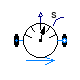Buildings.Fluid.Sensors.SpecificEntropyTwoPort

Ideal two port sensor for the specific entropy### Information

This model outputs the specific entropy of the passing fluid. The sensor is ideal, i.e., it does not influence the fluid. If the parameter `tau` is non-zero, then its output is computed using a first order differential equation. Setting `tau=0` is not recommend. See Buildings.Fluid.Sensors.UsersGuide for an explanation.

Extends from Buildings.Fluid.Sensors.BaseClasses.PartialDynamicFlowSensor (Partial component to model sensors that measure flow properties using a dynamic model), Modelica.Icons.RotationalSensor (Icon representing a round measurement device).

### Parameters

TypeNameDefaultDescription
replaceable package MediumPartialMediumMedium in the component
Timetau1Time constant at nominal flow rate [s]
Nominal condition
MassFlowRatem_flow_nominal Nominal mass flow rate, used for regularization near zero flow [kg/s]
MassFlowRatem_flow_small1E-4*m_flow_nominalFor bi-directional flow, temperature is regularized in the region |m_flow| < m_flow_small (m_flow_small > 0 required) [kg/s]
Initialization
InitinitTypeModelica.Blocks.Types.Init.I...Type of initialization (InitialState and InitialOutput are identical)
SpecificEntropys_startMedium.specificEntropy_pTX(p...Initial or guess value of output (= state) [J/(kg.K)]
Assumptions
BooleanallowFlowReversalsystem.allowFlowReversal= true to allow flow reversal, false restricts to design direction (port_a -> port_b)

### Connectors

TypeNameDescription
FluidPort_aport_aFluid connector a (positive design flow direction is from port_a to port_b)
FluidPort_bport_bFluid connector b (positive design flow direction is from port_a to port_b)
output RealOutputsSpecific entropy of the passing fluid [J/(kg.K)]

### Modelica definition

```model SpecificEntropyTwoPort
"Ideal two port sensor for the specific entropy"
extends Buildings.Fluid.Sensors.BaseClasses.PartialDynamicFlowSensor;
extends Modelica.Icons.RotationalSensor;
parameter Modelica.SIunits.SpecificEntropy s_start=
Medium.specificEntropy_pTX(p=Medium.p_default, T=Medium.T_default, X=Medium.X_default)
"Initial or guess value of output (= state)";
Modelica.Blocks.Interfaces.RealOutput s(final quantity="SpecificEntropy",
final unit="J/(kg.K)",
start=s_start)
"Specific entropy of the passing fluid";
protected
Modelica.SIunits.SpecificEntropy sMed(start=s_start)
"Medium entropy to which the sensor is exposed";
Medium.SpecificEntropy s_a_inflow
"Specific entropy of inflowing fluid at port_a";
Medium.SpecificEntropy s_b_inflow
"Specific entropy of inflowing fluid at port_b, or s_a_inflow if uni-directional flow";
initial equation
if dynamic then
der(s) = 0;
elseif initType == Modelica.Blocks.Types.Init.InitialState or
initType == Modelica.Blocks.Types.Init.InitialOutput then
s = s_start;
end if;
end if;
equation
if allowFlowReversal then
s_a_inflow = Medium.specificEntropy(state=
Medium.setState_phX(p=port_b.p, h=port_b.h_outflow, X=port_b.Xi_outflow));
s_b_inflow = Medium.specificEntropy(state=
Medium.setState_phX(p=port_a.p, h=port_a.h_outflow, X=port_a.Xi_outflow));
sMed = Modelica.Fluid.Utilities.regStep(
x=port_a.m_flow,
y1=s_a_inflow,
y2=s_b_inflow,
x_small=m_flow_small);
else
sMed = Medium.specificEntropy(state=
Medium.setState_phX(p=port_b.p, h=port_b.h_outflow, X=port_b.Xi_outflow));
s_a_inflow = sMed;
s_b_inflow = sMed;
end if;
// Output signal of sensor
if dynamic then
der(s) = (sMed-s)*k/tau;
else
s = sMed;
end if;
end SpecificEntropyTwoPort;
```

##Buildings.Fluid.Sensors.Temperature

Ideal one port temperature sensor### Information

This model outputs the temperature of the fluid connected to its port. The sensor is ideal, i.e., it does not influence the fluid.

Read the Buildings.Fluid.Sensors.UsersGuide prior to using this model with one fluid port.

Extends from Buildings.Fluid.Sensors.BaseClasses.PartialAbsoluteSensor (Partial component to model a sensor that measures a potential variable).

### Parameters

TypeNameDefaultDescription
replaceable package MediumPartialMediumMedium in the sensor

### Connectors

TypeNameDescription
FluidPort_aport
output RealOutputTTemperature in port medium [K]

### Modelica definition

```model Temperature "Ideal one port temperature sensor"
extends Buildings.Fluid.Sensors.BaseClasses.PartialAbsoluteSensor;

Modelica.Blocks.Interfaces.RealOutput T(final quantity="ThermodynamicTemperature",
final unit = "K",
min=0,
displayUnit = "degC")
"Temperature in port medium";

equation
T = Medium.temperature(state=Medium.setState_phX(
p=port.p, h=inStream(port.h_outflow), X=inStream(port.Xi_outflow)));
end Temperature;
```

##Buildings.Fluid.Sensors.TemperatureTwoPort

Ideal two port temperature sensor### Information

This model outputs the temperature of the medium in the flow between its fluid ports. The sensor does not influence the fluid. If the parameter `tau` is non-zero, then its output is computed using a first order differential equation. Setting `tau=0` is not recommend. See Buildings.Fluid.Sensors.UsersGuide for an explanation.

Extends from Buildings.Fluid.Sensors.BaseClasses.PartialDynamicFlowSensor (Partial component to model sensors that measure flow properties using a dynamic model).

### Parameters

TypeNameDefaultDescription
replaceable package MediumPartialMediumMedium in the component
Timetau1Time constant at nominal flow rate [s]
Nominal condition
MassFlowRatem_flow_nominal Nominal mass flow rate, used for regularization near zero flow [kg/s]
MassFlowRatem_flow_small1E-4*m_flow_nominalFor bi-directional flow, temperature is regularized in the region |m_flow| < m_flow_small (m_flow_small > 0 required) [kg/s]
Initialization
InitinitTypeModelica.Blocks.Types.Init.I...Type of initialization (InitialState and InitialOutput are identical)
TemperatureT_startMedium.T_defaultInitial or guess value of output (= state) [K]
Assumptions
BooleanallowFlowReversalsystem.allowFlowReversal= true to allow flow reversal, false restricts to design direction (port_a -> port_b)

### Connectors

TypeNameDescription
FluidPort_aport_aFluid connector a (positive design flow direction is from port_a to port_b)
FluidPort_bport_bFluid connector b (positive design flow direction is from port_a to port_b)
output RealOutputTTemperature of the passing fluid [K]

### Modelica definition

```model TemperatureTwoPort "Ideal two port temperature sensor"
extends Buildings.Fluid.Sensors.BaseClasses.PartialDynamicFlowSensor;
Modelica.Blocks.Interfaces.RealOutput T(final quantity="ThermodynamicTemperature",
final unit="K",
displayUnit = "degC",
min = 0,
start=T_start)
"Temperature of the passing fluid";
parameter Modelica.SIunits.Temperature T_start=Medium.T_default
"Initial or guess value of output (= state)";

protected
Medium.Temperature TMed(start=T_start)
"Medium temperature to which the sensor is exposed";
Medium.Temperature T_a_inflow "Temperature of inflowing fluid at port_a";
Medium.Temperature T_b_inflow
"Temperature of inflowing fluid at port_b, or T_a_inflow if uni-directional flow";
initial equation
if dynamic then
der(T) = 0;
elseif initType == Modelica.Blocks.Types.Init.InitialState or
initType == Modelica.Blocks.Types.Init.InitialOutput then
T = T_start;
end if;
end if;
equation
if allowFlowReversal then
T_a_inflow = Medium.temperature(state=
Medium.setState_phX(p=port_b.p, h=port_b.h_outflow, X=port_b.Xi_outflow));
T_b_inflow = Medium.temperature(state=
Medium.setState_phX(p=port_a.p, h=port_a.h_outflow, X=port_a.Xi_outflow));
TMed = Modelica.Fluid.Utilities.regStep(
x=port_a.m_flow,
y1=T_a_inflow,
y2=T_b_inflow,
x_small=m_flow_small);
else
TMed = Medium.temperature(state=
Medium.setState_phX(p=port_b.p, h=port_b.h_outflow, X=port_b.Xi_outflow));
T_a_inflow = TMed;
T_b_inflow = TMed;
end if;
// Output signal of sensor
if dynamic then
der(T) = (TMed-T)*k/tau;
else
T = TMed;
end if;
end TemperatureTwoPort;
```

##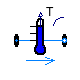Buildings.Fluid.Sensors.TemperatureWetBulbTwoPort

Ideal wet bulb temperature sensor### Information

This sensor outputs the wet bulb temperature of the medium in the flow between its fluid ports. The sensor is ideal, i.e., it does not influence the fluid. If the parameter `tau` is non-zero, then its output is computed using a first order differential equation. Setting `tau=0` is not recommend. See Buildings.Fluid.Sensors.UsersGuide for an explanation.

Extends from Buildings.Fluid.Sensors.BaseClasses.PartialDynamicFlowSensor (Partial component to model sensors that measure flow properties using a dynamic model).

### Parameters

TypeNameDefaultDescription
replaceable package MediumPartialMediumMedium in the component
Timetau1Time constant at nominal flow rate [s]
Nominal condition
MassFlowRatem_flow_nominal Nominal mass flow rate, used for regularization near zero flow [kg/s]
MassFlowRatem_flow_small1E-4*m_flow_nominalFor bi-directional flow, temperature is regularized in the region |m_flow| < m_flow_small (m_flow_small > 0 required) [kg/s]
Initialization
InitinitTypeModelica.Blocks.Types.Init.I...Type of initialization (InitialState and InitialOutput are identical)
TemperatureTWetBul_startMedium.T_defaultInitial or guess value of wet bulb temperature (used to compute initial output signal)) [K]
Assumptions
BooleanallowFlowReversalsystem.allowFlowReversal= true to allow flow reversal, false restricts to design direction (port_a -> port_b)

### Connectors

TypeNameDescription
FluidPort_aport_aFluid connector a (positive design flow direction is from port_a to port_b)
FluidPort_bport_bFluid connector b (positive design flow direction is from port_a to port_b)
output RealOutputTWet bulb temperature in port medium [K]

### Modelica definition

```model TemperatureWetBulbTwoPort "Ideal wet bulb temperature sensor"
extends Buildings.Fluid.Sensors.BaseClasses.PartialDynamicFlowSensor;
Modelica.Blocks.Interfaces.RealOutput T(
start=TWetBul_start,
final quantity="ThermodynamicTemperature",
final unit="K",
displayUnit = "degC") "Wet bulb temperature in port medium";
parameter Modelica.SIunits.Temperature TWetBul_start = Medium.T_default
"Initial or guess value of wet bulb temperature (used to compute initial output signal))";

protected
Medium.Temperature TMedWetBul(start=TWetBul_start)
"Medium wet bulb temperature to which the sensor is exposed";
Buildings.Utilities.Psychrometrics.TWetBul_TDryBulXi wetBulMod(
redeclare package Medium = Medium,
TWetBul(start=TWetBul_start)) "Block for wet bulb temperature";
Modelica.SIunits.SpecificEnthalpy h "Specific enthalpy";
Medium.MassFraction Xi[Medium.nXi]
"Species vector, needed because indexed argument for the operator inStream is not supported";
initial equation
// Initialization of wet bulb temperature
if dynamic then
der(T) = 0;
elseif initType == Modelica.Blocks.Types.Init.InitialState or
initType == Modelica.Blocks.Types.Init.InitialOutput then
T = TWetBul_start;
end if;
end if;
equation
if allowFlowReversal then
h  = Modelica.Fluid.Utilities.regStep(
x=port_a.m_flow,
y1=port_b.h_outflow,
y2=port_a.h_outflow,
x_small=m_flow_small);
Xi = Modelica.Fluid.Utilities.regStep(
x=port_a.m_flow,
y1=port_b.Xi_outflow,
y2=port_a.Xi_outflow,
x_small=m_flow_small);
else
h = port_b.h_outflow;
Xi = port_b.Xi_outflow;
end if;
// Compute wet bulb temperature
wetBulMod.TDryBul = Medium.T_phX(p=port_a.p, h=h, X=cat(1,Xi,{1-sum(Xi)}));
wetBulMod.Xi = Xi;
wetBulMod.p  = port_a.p;
TMedWetBul = wetBulMod.TWetBul;
// Output signal of sensor
if dynamic then
der(T) = (TMedWetBul-T)*k/tau;
else
T = TMedWetBul;
end if;
end TemperatureWetBulbTwoPort;
```

##Buildings.Fluid.Sensors.TraceSubstances

Ideal one port trace substances sensor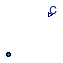### Information

This model outputs the trace substances contained in the fluid connected to its port. The sensor is ideal, i.e., it does not influence the fluid.

Read the Buildings.Fluid.Sensors.UsersGuide prior to using this model with one fluid port.

Extends from Buildings.Fluid.Sensors.BaseClasses.PartialAbsoluteSensor (Partial component to model a sensor that measures a potential variable), Modelica.Icons.RotationalSensor (Icon representing a round measurement device).

### Parameters

TypeNameDefaultDescription
replaceable package MediumPartialMediumMedium in the sensor
StringsubstanceName"CO2"Name of trace substance

### Connectors

TypeNameDescription
FluidPort_aport
output RealOutputCTrace substance in port medium

### Modelica definition

```model TraceSubstances "Ideal one port trace substances sensor"
extends Buildings.Fluid.Sensors.BaseClasses.PartialAbsoluteSensor;
extends Modelica.Icons.RotationalSensor;
parameter String substanceName = "CO2" "Name of trace substance";

Modelica.Blocks.Interfaces.RealOutput C(min=0)
"Trace substance in port medium";

protected
parameter Real s[Medium.nC](each fixed=false)
"Vector with zero everywhere except where species is";
initial algorithm
for i in 1:Medium.nC loop
if ( Modelica.Utilities.Strings.isEqual(string1=Medium.extraPropertiesNames[i],
string2=substanceName,
caseSensitive=false)) then
s[i] :=1;
else
s[i] :=0;
end if;
end for;
assert(abs(1-sum(s)) < 1E-4, "Trace substance '" + substanceName + "' is not present in medium '"
+ Medium.mediumName + "'.\n"
+ "Check sensor parameter and medium model.");
equation
// We obtain the species concentration with a vector multiplication
// because Dymola 7.3 cannot find the derivative in the model
// Buildings.Examples.VAVSystemCTControl.mo
// if we set C = CVec[ind];
C = s*inStream(port.C_outflow);
end TraceSubstances;
```

##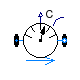Buildings.Fluid.Sensors.TraceSubstancesTwoPort

Ideal two port sensor for trace substance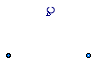### Information

This model outputs the trace substance of the passing fluid. The sensor is ideal, i.e., it does not influence the fluid. If the parameter `tau` is non-zero, then its output is computed using a first order differential equation. Setting `tau=0` is not recommend. See Buildings.Fluid.Sensors.UsersGuide for an explanation.

Extends from Buildings.Fluid.Sensors.BaseClasses.PartialDynamicFlowSensor (Partial component to model sensors that measure flow properties using a dynamic model), Modelica.Icons.RotationalSensor (Icon representing a round measurement device).

### Parameters

TypeNameDefaultDescription
replaceable package MediumPartialMediumMedium in the component
Timetau1Time constant at nominal flow rate [s]
StringsubstanceName"CO2"Name of trace substance
Nominal condition
MassFlowRatem_flow_nominal Nominal mass flow rate, used for regularization near zero flow [kg/s]
MassFlowRatem_flow_small1E-4*m_flow_nominalFor bi-directional flow, temperature is regularized in the region |m_flow| < m_flow_small (m_flow_small > 0 required) [kg/s]
Initialization
InitinitTypeModelica.Blocks.Types.Init.I...Type of initialization (InitialState and InitialOutput are identical)
RealC_start0Initial or guess value of output (= state)
Assumptions
BooleanallowFlowReversalsystem.allowFlowReversal= true to allow flow reversal, false restricts to design direction (port_a -> port_b)

### Connectors

TypeNameDescription
FluidPort_aport_aFluid connector a (positive design flow direction is from port_a to port_b)
FluidPort_bport_bFluid connector b (positive design flow direction is from port_a to port_b)
output RealOutputCTrace substance of the passing fluid

### Modelica definition

```model TraceSubstancesTwoPort
"Ideal two port sensor for trace substance"
extends Buildings.Fluid.Sensors.BaseClasses.PartialDynamicFlowSensor;
extends Modelica.Icons.RotationalSensor;
Modelica.Blocks.Interfaces.RealOutput C(min=0,
start=C_start)
"Trace substance of the passing fluid";
parameter String substanceName = "CO2" "Name of trace substance";
parameter Real C_start(min=0) = 0
"Initial or guess value of output (= state)";

protected
Real CMed(min=0, start=C_start, nominal=sum(Medium.C_nominal))
"Medium trace substance to which the sensor is exposed";
parameter Real s[Medium.nC](each fixed=false)
"Vector with zero everywhere except where the trace substance is";
initial algorithm
for i in 1:Medium.nC loop
if ( Modelica.Utilities.Strings.isEqual(string1=Medium.extraPropertiesNames[i],
string2=substanceName,
caseSensitive=false)) then
s[i] :=1;
else
s[i] :=0;
end if;
end for;
assert(abs(1-sum(s))<1E-4, "Trace substance '" + substanceName + "' is not present in medium '"
+ Medium.mediumName + "'.\n"
+ "Check sensor parameter and medium model.");
initial equation
if dynamic then
der(C) = 0;
elseif initType == Modelica.Blocks.Types.Init.InitialState or
initType == Modelica.Blocks.Types.Init.InitialOutput then
C = C_start;
end if;
end if;
equation
if allowFlowReversal then
CMed = Modelica.Fluid.Utilities.regStep(
x=port_a.m_flow,
y1=s*port_b.C_outflow,
y2=s*port_a.C_outflow,
x_small=m_flow_small);
else
CMed = s*inStream(port_b.C_outflow);
end if;
// Output signal of sensor
if dynamic then
der(C) = (CMed-C)*k/tau;
else
C = CMed;
end if;
end TraceSubstancesTwoPort;
```

##Buildings.Fluid.Sensors.VolumeFlowRate

Ideal sensor for volume flow rate### Information

This model outputs the volume flow rate flowing from `port_a` to `port_b`. The sensor is ideal, i.e., it does not influence the fluid. If the parameter `tau` is non-zero, then the measured density that is used to convert the mass flow rate into volumetric flow rate is computed using a first order differential equation. Setting `tau=0` is not recommend. See Buildings.Fluid.Sensors.UsersGuide for an explanation.

Extends from Buildings.Fluid.Sensors.BaseClasses.PartialDynamicFlowSensor (Partial component to model sensors that measure flow properties using a dynamic model), Modelica.Icons.RotationalSensor (Icon representing a round measurement device).

### Parameters

TypeNameDefaultDescription
replaceable package MediumPartialMediumMedium in the component
Timetau1Time constant at nominal flow rate [s]
Nominal condition
MassFlowRatem_flow_nominal Nominal mass flow rate, used for regularization near zero flow [kg/s]
MassFlowRatem_flow_small1E-4*m_flow_nominalFor bi-directional flow, temperature is regularized in the region |m_flow| < m_flow_small (m_flow_small > 0 required) [kg/s]
Initialization
InitinitTypeModelica.Blocks.Types.Init.I...Type of initialization (InitialState and InitialOutput are identical)
Densityd_startMedium.density(Medium.setSta...Initial or guess value of density [kg/m3]
TemperatureT_startMedium.T_defaultTemperature used to compute d_start [K]
Pressurep_startMedium.p_defaultPressure used to compute d_start [Pa]
MassFractionX_start[Medium.nX]Medium.X_defaultMass fraction used to compute d_start 
Assumptions
BooleanallowFlowReversalsystem.allowFlowReversal= true to allow flow reversal, false restricts to design direction (port_a -> port_b)

### Connectors

TypeNameDescription
FluidPort_aport_aFluid connector a (positive design flow direction is from port_a to port_b)
FluidPort_bport_bFluid connector b (positive design flow direction is from port_a to port_b)
output RealOutputV_flowVolume flow rate from port_a to port_b [m3/s]

### Modelica definition

```model VolumeFlowRate "Ideal sensor for volume flow rate"
extends Buildings.Fluid.Sensors.BaseClasses.PartialDynamicFlowSensor;
extends Modelica.Icons.RotationalSensor;
parameter Medium.Density
d_start=Medium.density(Medium.setState_pTX(p_start, T_start, X_start))
"Initial or guess value of density";
parameter Modelica.SIunits.Temperature T_start=Medium.T_default
"Temperature used to compute d_start";
parameter Modelica.SIunits.Pressure p_start=Medium.p_default
"Pressure used to compute d_start";
parameter Modelica.SIunits.MassFraction X_start[Medium.nX]=Medium.X_default
"Mass fraction used to compute d_start";
Modelica.Blocks.Interfaces.RealOutput V_flow(final quantity="VolumeFlowRate",
final unit="m3/s")
"Volume flow rate from port_a to port_b";
protected
Medium.Density dMed(start=d_start)
"Medium temperature to which the sensor is exposed";

Medium.Density d_a_inflow(start=d_start)
"Density of inflowing fluid at port_a";
Medium.Density d_b_inflow(start=d_start)
"Density of inflowing fluid at port_b, or rho_a_inflow if uni-directional flow";
Medium.Density d(start=d_start) "Density of the passing fluid";
initial equation
if dynamic then
der(d) = 0;
elseif initType == Modelica.Blocks.Types.Init.InitialState or
initType == Modelica.Blocks.Types.Init.InitialOutput then
d = d_start;
end if;
end if;
equation
if allowFlowReversal then
d_a_inflow = Medium.density(state=
Medium.setState_phX(p=port_b.p, h=port_b.h_outflow, X=port_b.Xi_outflow));
d_b_inflow = Medium.density(state=
Medium.setState_phX(p=port_a.p, h=port_a.h_outflow, X=port_a.Xi_outflow));
dMed = Modelica.Fluid.Utilities.regStep(
x=port_a.m_flow,
y1=d_a_inflow,
y2=d_b_inflow,
x_small=m_flow_small);
else
dMed = Medium.density(state=Medium.setState_phX(
p=port_b.p,
h=port_b.h_outflow,
X=port_b.Xi_outflow));
d_a_inflow = dMed;
d_b_inflow = dMed;
end if;
// Output signal of density sensor that is used to compute
// the volume flow rate
if dynamic then
der(d) = (dMed-d)*k/tau;
else
d = dMed;
end if;
// Volume flow rate
V_flow = port_a.m_flow/d;
end VolumeFlowRate;
```

Automatically generated Thu Oct 24 15:09:47 2013.